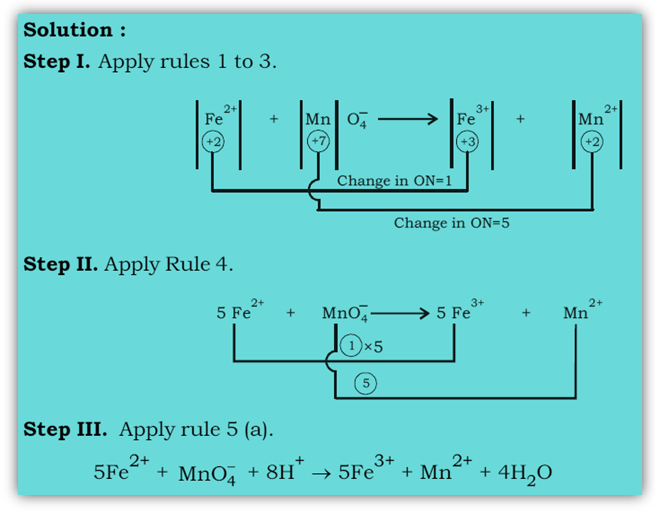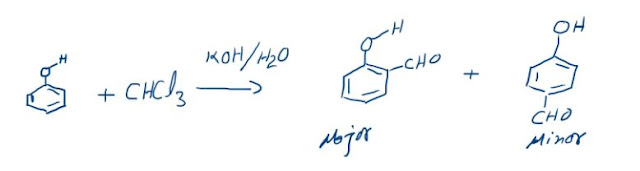## Methods used to balance redox reactions:

### (2)Half Reaction Method

1. Oxidation Number Method
The various steps involved in the balancing of redox equations by oxidation number method are:
Step1. Write the skeletal equation of all the reactants and products of the reaction.
Step2. Indicate the oxidation number of each element above its symbol and identify the elements which undergo a change in the oxidation number (O.N.).
Step3. Calculate the increase or decrease in oxidation number per atom and identify the Oxidising and reducing agents. If more than one atom of the same element is involved, find out the total increase or decrease in O.N. by multiplying this increase or decrease in O.N. per atom by the number of atoms undergoing that change.
Step4. Multiply the formulae of the Oxidising and the reducing agents by suitable integers so as to equalize the total increase or decrease in oxidation number as calculated in step 3.
Step5. Balance all atoms other than H and O.
Step6. Finally balance H and O atoms by adding H2O molecules using hit and trial method. Step 7. In case of ionic reactions,
(a)For acidic medium. First balance O atoms by adding H2O molecules to whatever side deficient in O atoms and then balance H atoms by adding H+ ions to whatever side deficient in H atoms.
(b) For basic medium. First balance O atoms by adding H2O molecules to whatever side deficient in O atoms. The H atoms are then balanced by adding H2O molecules equal in number to the deficiency of H atoms and an equal number of OH– ions are added to the opposite side of the equation. Remove the duplication, if any.
Example.
Balance the ionic equation: Fe2+ + MnO4- ----> Fe3+ + Mn2+ (acidic medium)### Half Reaction Method:

In this method the two half equations are balanced separately and then added together to give balanced equation.
Example.
Balance: I2 + S2O23- I - + S4O26-
First, separate oxidation and reduction Processes
S2O32 - S4O26- (oxd.)
I2 I (Reduction)
Now, balance the number of each atom like I and S, undergoing reduction and oxidation and then add electrons to the electrically positive side of the equation to balance the charge on both sides of the equation.
2S2O32 - S4 O62- + 2e
I2 + 2e - 2I-
Each balanced half-reaction involves two electrons. Add them. Electrons get cancelled.
I2 + 2 S2O23 - S4O26- + 2I-
The formula-unit equation may now be written as
I2 + 2 Na2S2O3 Na2S4O6 + 2 NaI
Here, Na+ ions are ‘spectator’ ions.
In this example, oxygen atoms are automatically balanced. In case they are not, the rules given in the ‘Oxidation-Number-Change Method’ have to be followed to balance atoms other than those undergoing a change in oxidation number.

### The Reimer-Tiemann reaction: Reaction and its Mechanism

Reimer-Tiemann Reaction Phenols on reaction with chloroform in the presence of sodium hydroxide (or potassium hydroxide) solution give hydro...